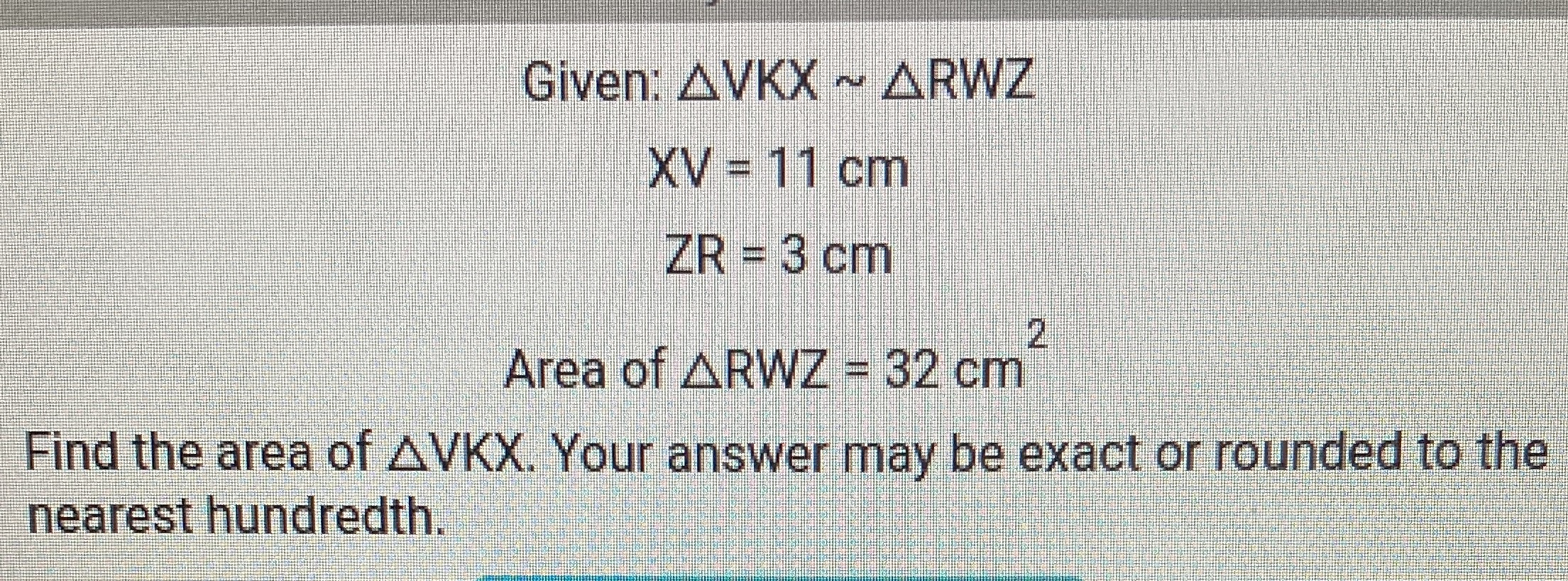### Still have math questions?

Trigonometry
QuestionGiven: $$\triangle V K X \sim \triangle R W Z$$

$$X V = 11 cm$$

$$Z R = 3 cm$$

Area of $$\triangle R W Z = 32 cm ^ { 2 }$$